# Combination of Resistors

(This article was translated from Japanese to English using DeepL.)

Ohm’s law is valid for a single resistor. When there are multiple resistors in a circuit, “Ohm’s law is used for as many resistors as there are resistors” as a rule.
When you see a circuit that contains three resistors, think, “I’m going to use Ohm’s law three times.”

So if 200 resistors are included, do we use Ohm’s law 200 times?
Yes, use 200 times.

Well, I think there are at most 4 problems in physics, so you don’t have to worry about such a huge amount of calculations. Still, when the number of problems is large, it is indeed a hassle, isn’t it? Is there any better way?

## Idea of Combination of Resistors

See the figure below.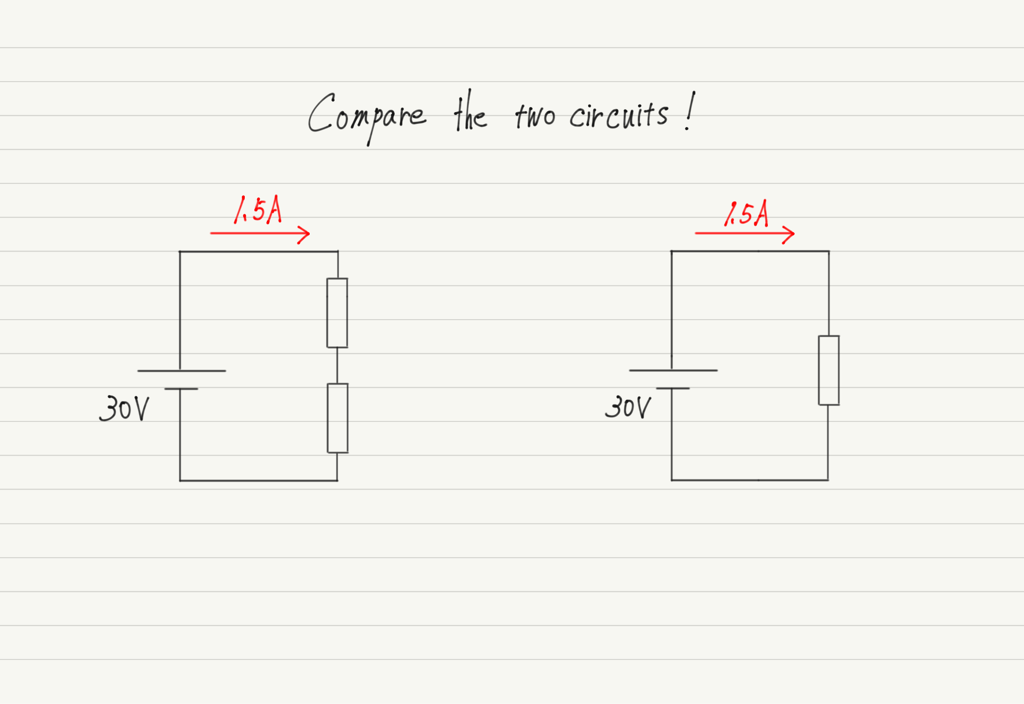Although they are different circuits, they are identical in that “when a 30 V battery is connected, a current of 1.5 A flows.

Since the same voltage was applied and the same current flowed, we can say that the two resistors in the left circuit and the resistor in the right circuit work in the same way.

In this way, multiple resistors can be grouped together and replaced with a single resistor that performs the same function (Combination of Resistors), which makes calculations much easier.
Because with one resistor, Ohm’s law only needs to be used once!

The problem is how many ohms of resistance to replace, which can be determined from the original resistors.
Let’s get right to the combination of resistors calculations!

## Combination of resistors calculation (series connection)

First, as before, let us compare a circuit with two resistors and a circuit in which they are replaced by a combination of resistors.
In each of these, we use Ohm’s law to calculate.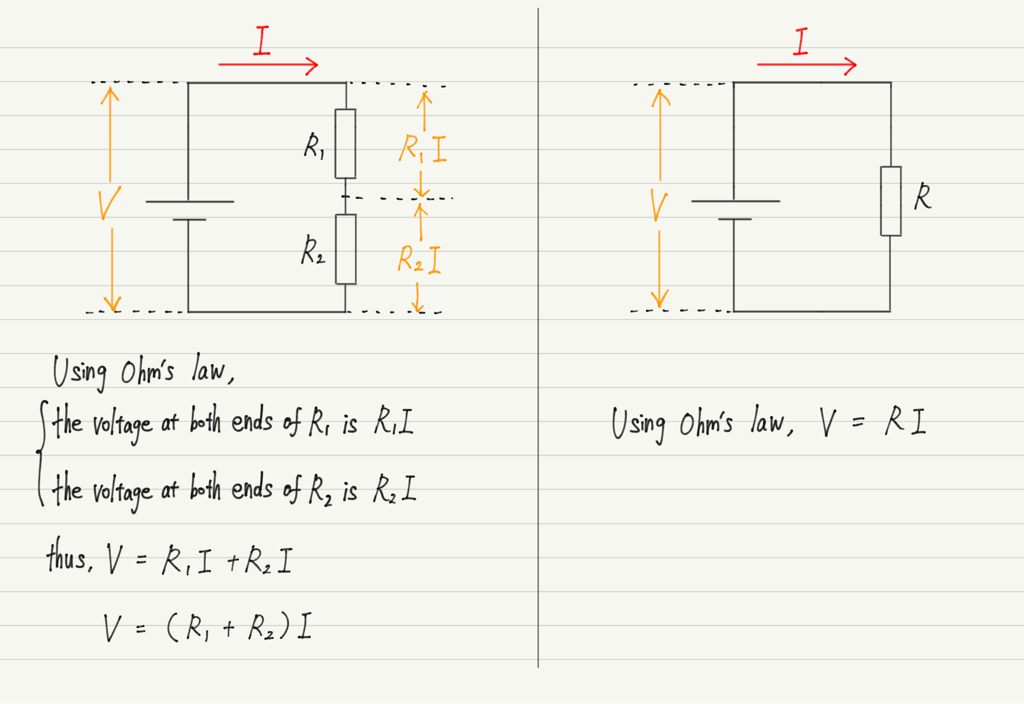Although the number of resistors in these two circuits is different, the equations must be the same because they are replaced by “resistors that perform the same function”.
Compare the two equations.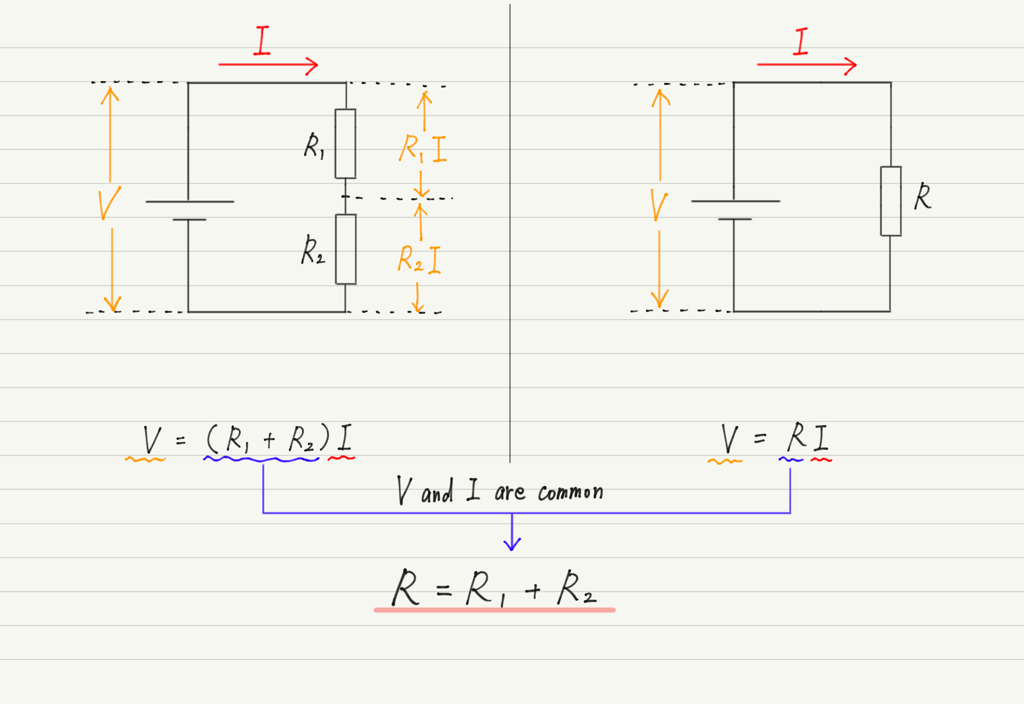Thus, if the resistors are connected in series, the combination of resistors value can be obtained by simply adding the values of the individual resistors.
If there are three or more resistors, just add them.

## Combination of resistors calculation (parallel connection)

As in the case of series connection, let’s use Ohm’s law in each of these calculations.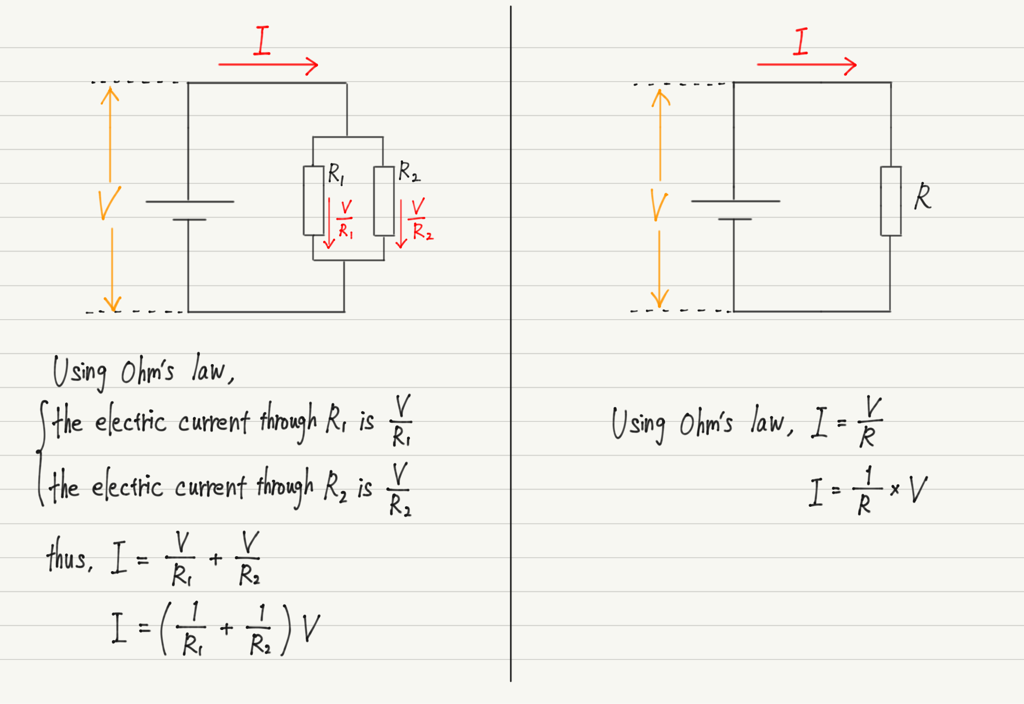Compare the two equations.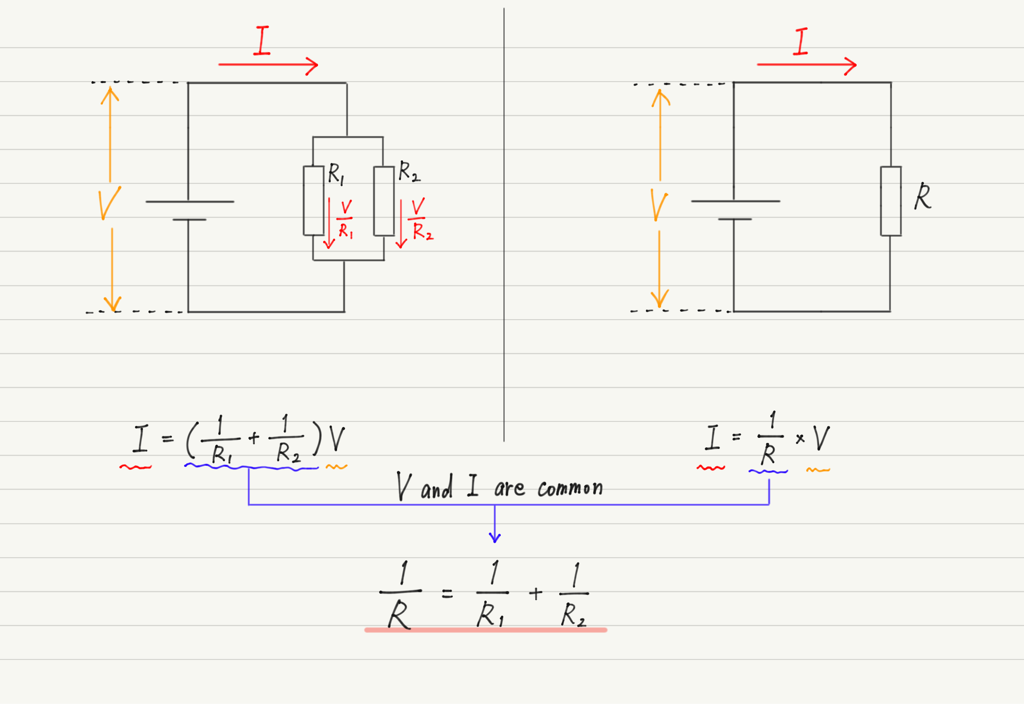In the case of parallel connection, the reciprocal of each resistance is added to the reciprocal of the combination of resistors.
If there are three or more resistors, just take the reciprocal and add them.

## Combination of resistors is not universal

Finally, try the exercise.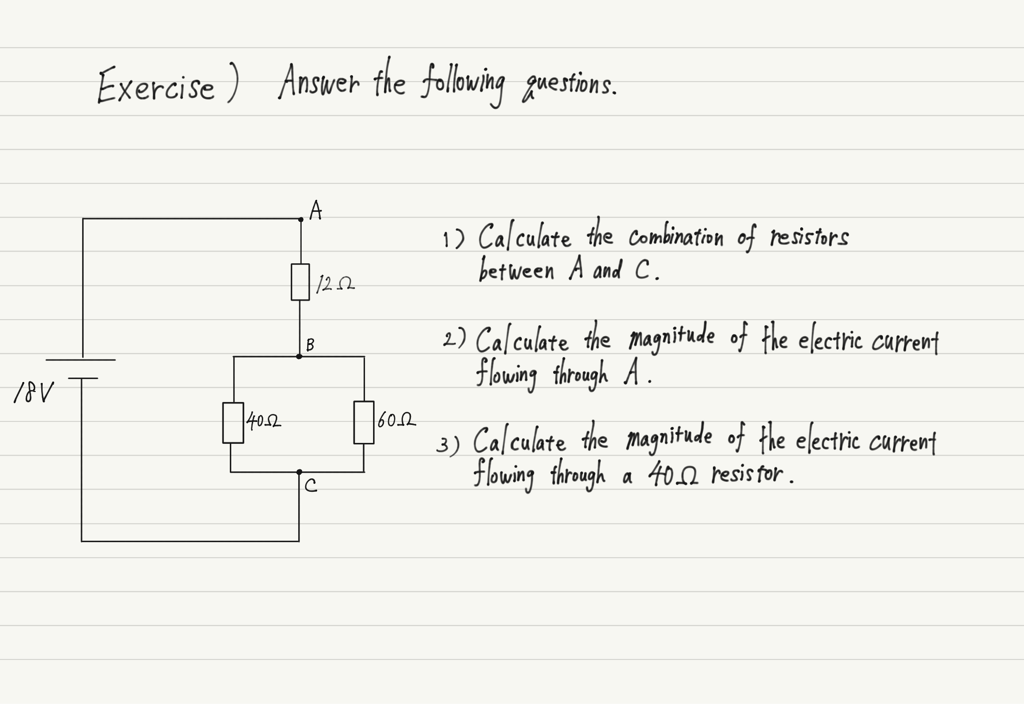The answer is below.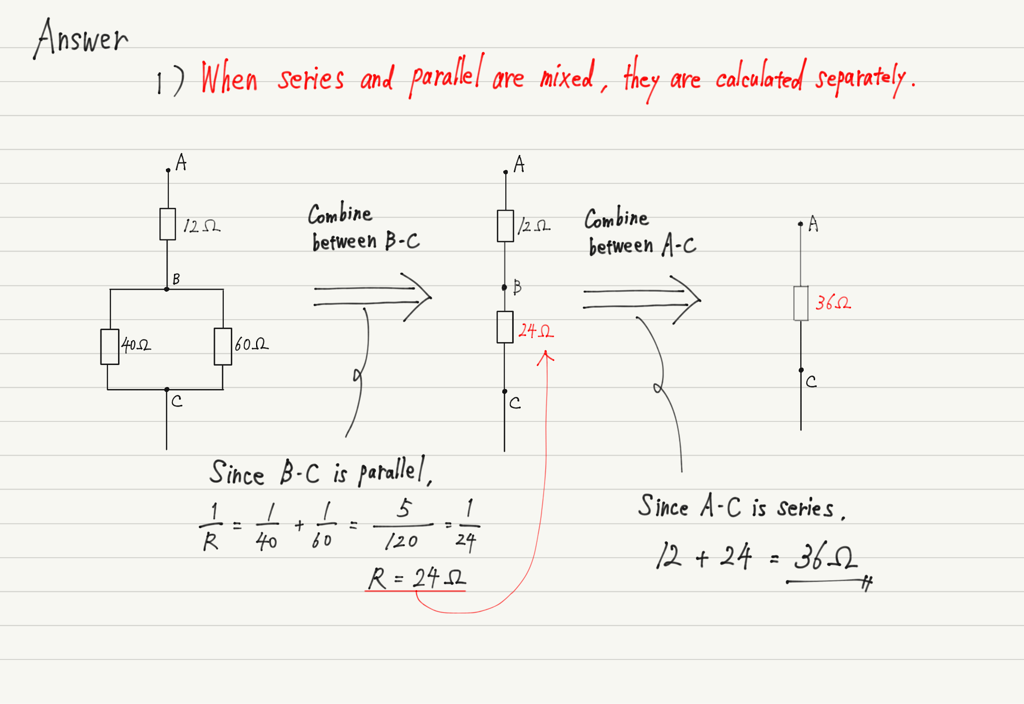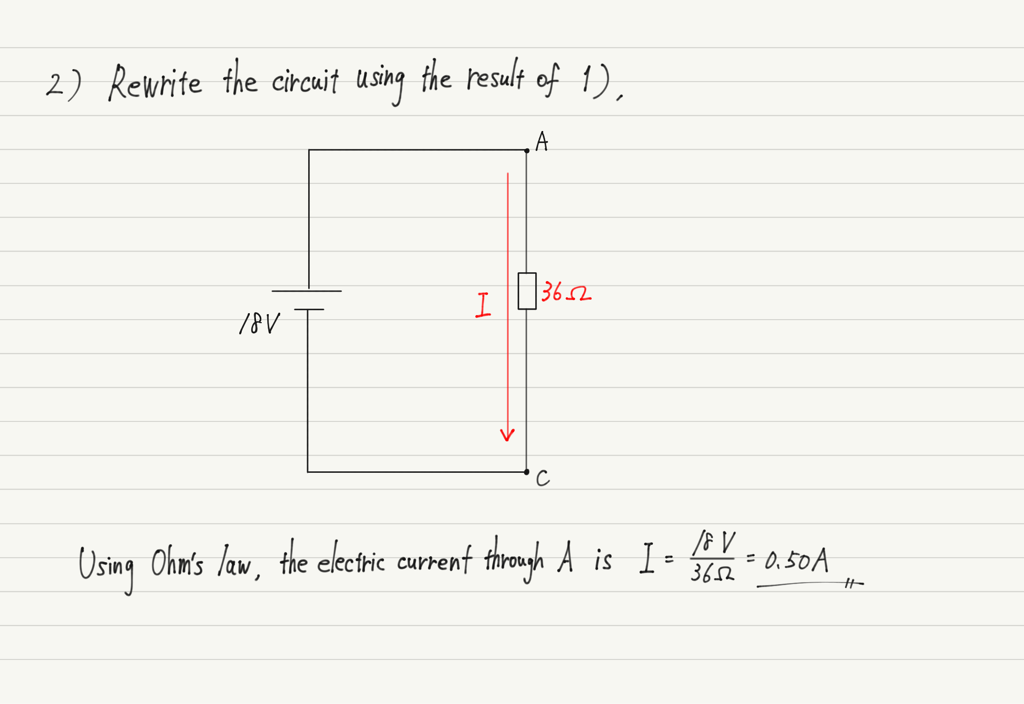The last problem cannot be solved using combination of resistors.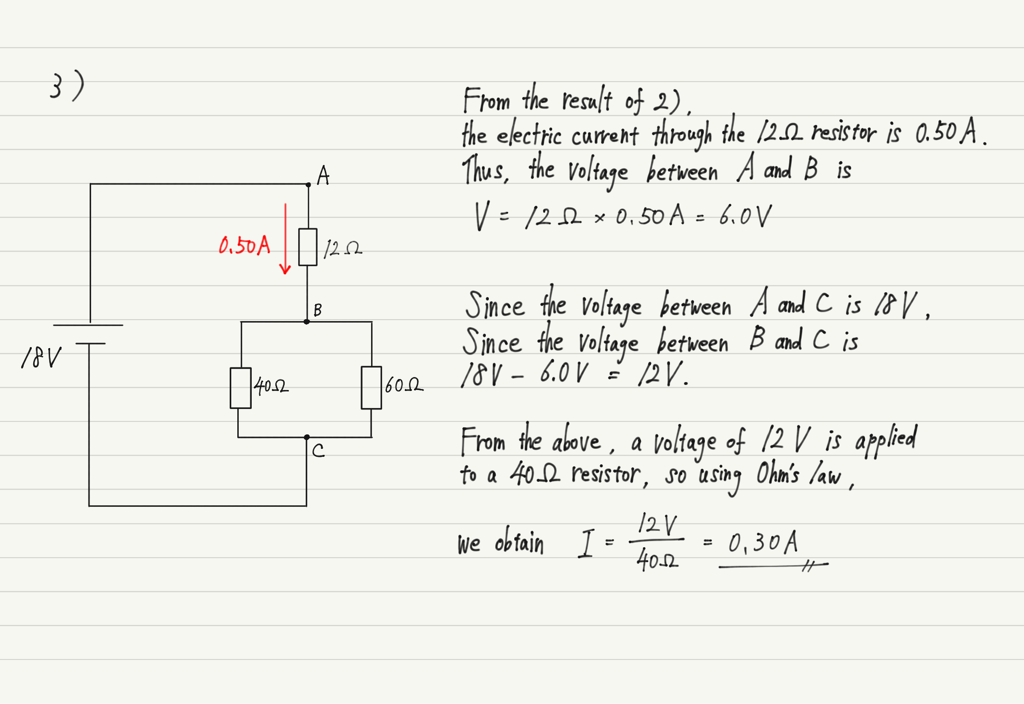Combination of resistors are a great help when calculating entire circuits, but they are useless for calculations on individual resistors.

Instead of using a combination of resistors for everything, be flexible and determine whether it is better to use a combination of resistors or not!

## Summary of this lecture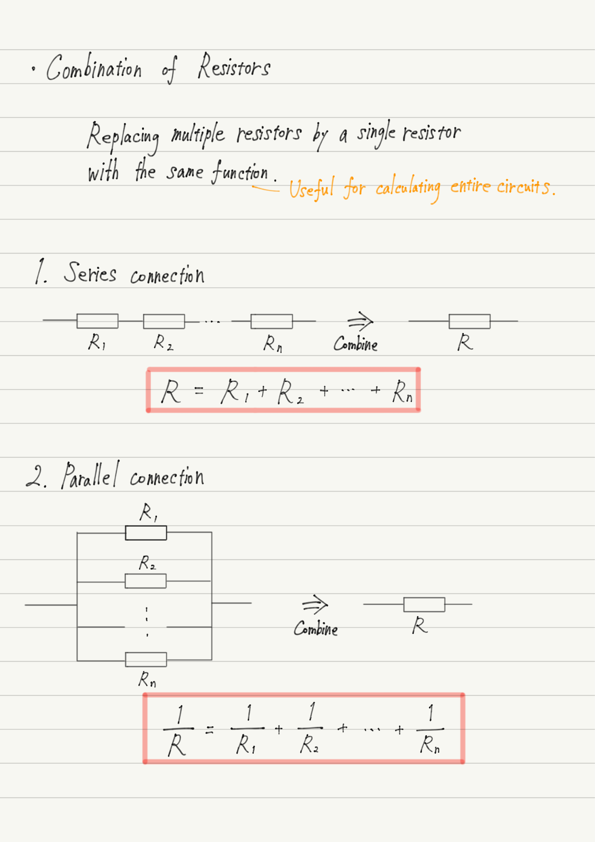error: Content is protected !!